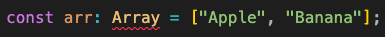# Array Types in TypeScript

Last updated on 9 Jan, 2023

Just like other data types, TypeScript can define the type of an array using different techniques.

## Inferred Array Type

Here is a normal array variable which is initialized with an array.

``const fruits = ["Apple", "Banana"];``

Here is the type automatically inferred by TypeScript from the assigned array.

``const fruits: string[];``

## Mixed Data Types

Let us have an array that has elements of different types.

``const fruits = ["Apple", 23];``

Now the inferred type for `fruits` is:

``const fruits: (string | number)[];``

It says that the array can contain either a string value or a number value.

## Set Types Explicitly

Say, we need to declare a variable `marks` that will contain an array of marks which are numbers. We can declare the variable with its type like below:

``let marks: number[];``

Then, later we can assign a number array to `marks`.

``marks = [23, 45];``

What if we assign a string array to `marks`? TypeScript can throw an error.--- ○ ---